Home | | Gas Dynamics and Jet Propulsion | Rayleigh line (or) curve

# Rayleigh line (or) curve

The frictionless flow of a perfect gas through a constant area duct in which heat transfer to or from the gas is the dominant factor bringing about changes in the flow is referred to as Rayleigh flow or diabetic flow.

Rayleigh line (or) curve

The friction less flow of a perfect gas through a constant area duct in which heat transfer to or from the gas is the dominant factor bringing about changes in the flow is referred to as Rayleigh flow or diabetic flow. In thermodynamic coordinates, the Rayleigh flow process can be described by a curve known as Rayleigh line and is defined as the locus of quasi- static thermodynamic state points traced during the flow. The Rayleigh line satisfies the equation of state along with simple forms of continuity and momentum equation.

Governing Equations

In order to formulate the equation for the Rayleigh line, let us consider steady flow of a perfect gas through a constant area passage in which transfer of heat with the surroundings is the major factor responsible for changes in fluid properties. The simple form of continuity equation for steady one dimensional flow in a constant area duct isWhere

G- Mass flow density.

c- Velocity of sound.

p- Density of fluid.

Equation 1 may be used for representing Rayleigh line on the h- s diagram, as illustrated in fig shown in below. In general, most of the fluids in practical use have Rayleigh curves of the general form shown in fig.The portion of the Rayleigh curve above the point of maximum entropy usually represents subsonic flow (M<1) and the portion below the maximum entropy point represents supersonic flow (M>1).

An entropy increases due to heat addition and entropy decreases due to heat rejection. Therefore, the Mach number is increased by heating and decreased by cooling at subsonic speeds. On the other hand, the Mach number is decreased by heating and increased by cooling at supersonic speeds. Therefore, like friction, heat addition also tends to make the Mach number in the duct approach unity. Cooling causes the Mach number to change in the direction away from unity.

Rayleigh Flow

Rayleigh flow refers to adiabetic flow through a constant area duct where the effect of heat addition or rejection is considered. Compressibility effects often come into consideration, although the Rayleigh flow model certainly also applies to incompressible flow. For this model, the duct area remains constant and no mass is added within the duct. Therefore, unlike Fanno flow, the stagnation temperature is a variable. The heat addition causes a decrease in stagnation pressure which is known as the Rayleigh effect and is critical in the design of combustion systems. Heat addition will cause both supersonic and subsonic Mach numbers to approach Mach 1, resulting in choked flow. Conversely, heat rejection decreases a subsonic Mach number and increases a supersonic Mach number along the duct. It can be shown that for calorically perfect flows the maximum entropy occurs at M = 1. Rayleigh flow is named after John Strutt, 3rd Baron Rayleigh.

TheoryThe Rayleigh flow model begins with a differential equation that relates the change in Mach number with the change in stagnation temperature, T0. The differential

equation is shown below.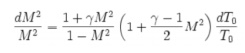Solving the differential equation leads to the relation shown below, where T0* is the stagnation temperature at the throat location of the duct which is required for thermally choking the flow.Fundamental Equations

The following fundamental equations will be used to determine the variation of flow parameters in Rayleigh flows.

Continuity equation

We know that

Mass flow rate,Where ;-

C1 –Velocity of fluid at inlet-m/s

C2 –Velocity of fluid at outlet-m/s

- Density of fluid at inlet-kg/m3

- Density of fluid at out let-kg/m3

Momentum equation

Momentum equation between state 1nad 2 is given by p1 A mc1 p2 A mc2

P1 A P2 A mc2 mc1

P1 P2 A m c2 c1Ø Mach Number

The Mach number at the two states are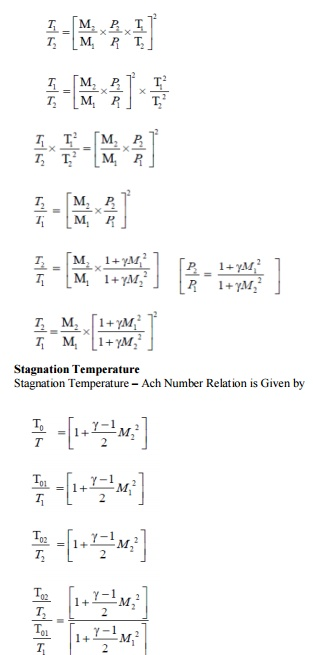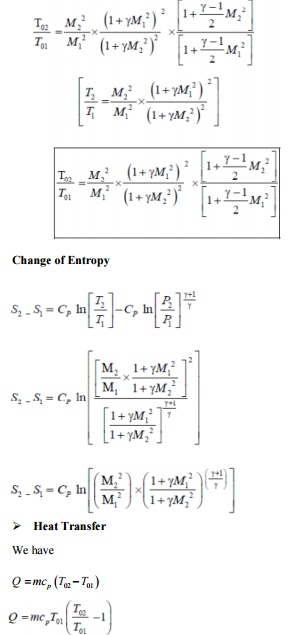Stagnation Pressue

Static Temperature

Stagnation Temperature

Expression for Heat Transfer we have

Q  mcp T02  T01

the condition 1 is fixed but the value of T02 attains its maximum when T02 = T0*

Problems based on Rayleigh flow

1.The condition of gas in a combustion chamber at entry are M1=0.28, T01=380 K, P01=4.9 bar. The heat supplied in the combustion chamber is 620 kJ/kg.Determine Mach number, pressure and temperature of the gas at exit and also determine the stagnation pressure loss during heating. Take γ= 1.3, cp=1.22 kJ/Kg K.

Given,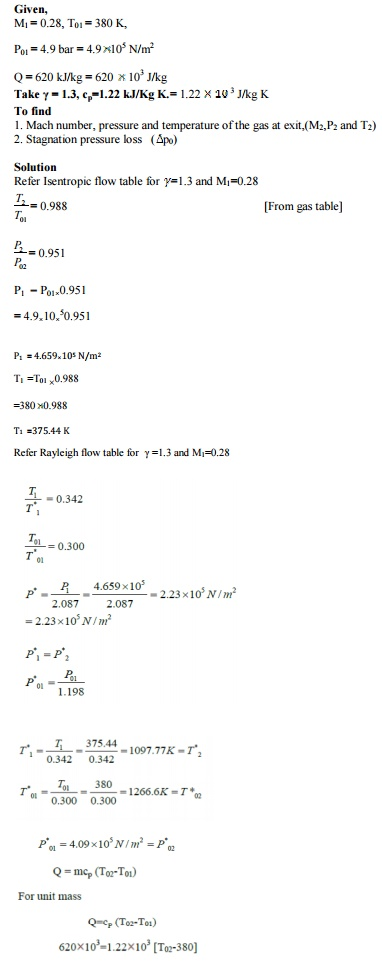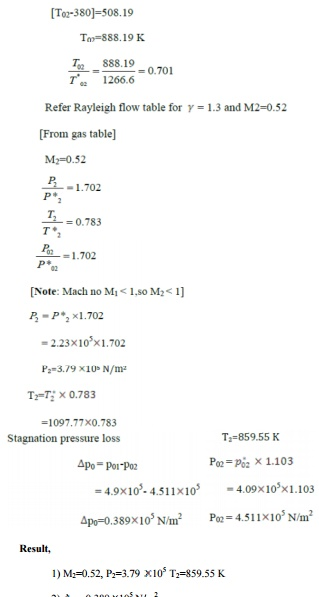2.A gas (γ=1.3 and R = 0.46 KJ / Kg K) at a pressure of 70 Kpa and temperature of 295 K enters a combustion chamber at a velocity of 75 m / sec. The heat supplied in a combustion chamber is 1250 KJ / Kg .Determine the Mach number, pressure and temperature of gas at exit.

Given:γ=1.3: R = 0.46 KJ / Kg K -1= 70 Kpa : T1 =295 K C1 = 75 m/sec: Q = 1250 KJ / Kg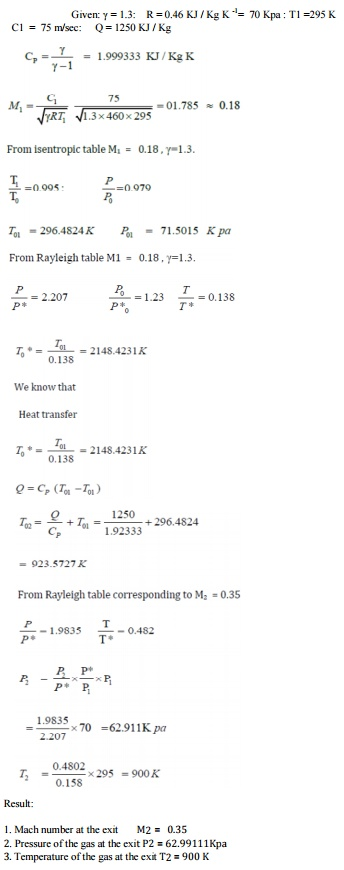Study Material, Lecturing Notes, Assignment, Reference, Wiki description explanation, brief detail
Mechanical : Gas Dynamics and Jet Propulsion : Flow Through Ducts : Rayleigh line (or) curve |